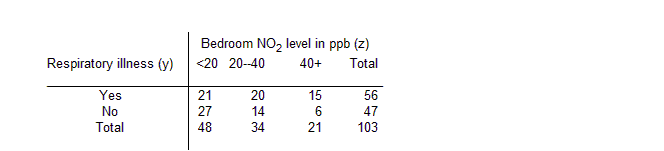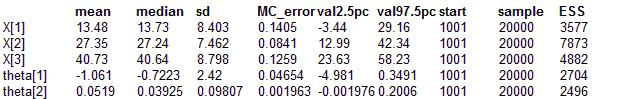#### Air: Berkson measurement error

Whittemore and Keller (1988) use an approximate maximum likelihood approach to analyse the data shown below on reported respiratory illness versus exposure to nitrogen dioxide (NO2) in 103 children. Stephens and Dellaportas (1992) later use Bayesian methods to analyse the same data.A discrete covariate zj (j = 1,2,3) representing NO2 concentration in the child's bedroom classified into 3 categories is used as a surrogate for true exposure. The nature of the measurement error relationship associated with this covariate is known precisely via a calibration study, and is given by
xj = α + β zj + εj

where α = 4.48, β = 0.76 and εj is a random element having normal distribution with zero mean and variance σ2 (= 1/τ) = 81.14. Note that this is a Berkson (1950) model of measurement error, in which the true values of the covariate are expressed as a function of the observed values. Hence the measurement error is independent of the latter, but is correlated with the true underlying covariate values. In the present example, the observed covariate zj takes values 10, 30 or 50 for j = 1, 2, or 3 respectively (i.e. the mid-point of each category), whilst xj is interpreted as the "true average value" of NO2 in group j. The response variable is binary, reflecting presence/absence of respiratory illness, and a logistic regression model is assumed. That is

yj ~ Binomial(pj, nj)
logit(pj) = θ1 + θ2 xj
where pj is the probability of respiratory illness for children in the jth exposure group. The regression coefficients θ1 and θ2 are given vague independent normal priors. The graphical model is shown below:
model
{
for(j in 1 : J) {
y[j] ~ dbin(p[j], n[j])
logit(p[j]) <- theta + theta * X[j]
X[j] ~ dnorm(mu[j], tau)
mu[j] <- alpha + beta * Z[j]
}
theta ~ dnorm(0.0, 0.001)
theta ~ dnorm(0.0, 0.001)
}

##### Data
``` list(J = 3, y = c(21, 20, 15), n = c(48, 34, 21), Z = c(10, 30, 50), tau = 0.01234, alpha = 4.48, beta = 0.76) ```
##### Inits for chain 1
```    list(theta = c(0.0, 0.0), X = c(0.0, 0.0, 0.0)) ```

##### Inits for chain 2
``` list(theta = c(1.0, 1.0), X = c(10.0, 30.0, 40.0)) ```
Results